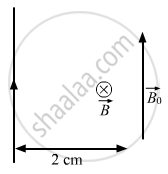Department of Pre-University Education, KarnatakaPUC Karnataka Science Class 12
Advertisement Remove all ads

# A Long, Straight Wire Carrying a Current of 30 a is Placed in an External, Uniform Magnetic Field of 4.0 × 10−4 T Parallel to the Current. Find the Magnitude of the Resultant Magnetic Field - Physics

Short Note

A long, straight wire carrying a current of 30 A is placed in an external, uniform magnetic field of 4.0 × 10−4 T parallel to the current. Find the magnitude of the resultant magnetic field at a point 2.0 cm away from the wire.

Advertisement Remove all ads

#### Solution

Given:
Uniform magnetic field, B0 = 4.0 × 10−4
Magnitude of current, I = 30 A
Separation of the point from the wire, d = 0.02 m
Thus, the magnetic field due to current in the wire is given by

$B = \frac{\mu_0 I}{2\pi d}$

$= \frac{2 \times {10}^{- 7} \times 30}{0 . 02}$

$= 3 \times {10}^{- 4} T$B0 is perpendicular to B (as shown in the figure).
∴ Resultant magnetic field

$B_{net} = \sqrt{B^2 + {B_0}^2}$

$= \sqrt{(4 \times {10}^{- 4} )^2 + (3 \times {10}^{- 4} )^2}$

$= 5 \times {10}^{- 4} T$

Concept: Force on a Moving Charge in Uniform Magnetic and Electric Fields
Is there an error in this question or solution?
Advertisement Remove all ads

#### APPEARS IN

HC Verma Class 11, Class 12 Concepts of Physics Vol. 2
Chapter 13 Magnetic Field due to a Current
Q 7 | Page 250
Advertisement Remove all ads
Advertisement Remove all ads
Share
Notifications

View all notifications

Forgot password?